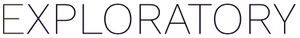# Excel: When cell format is text, imported numeric value has rounding error

#1

Assume we have an xlsx file that contains data like this. Value is a text column.

When we import this data to Exploratory, Exploratory Desktop shows value to something different (for example 132834.3 vs 132834.29999999999)

As you can see in below example, the value 132834.3 in Excel is shown as 132834.29999999999 in Exploratory Desktop where we expect It to be the same as Excel (i.e. 132834.3)

This is a known issue for `readxl` R package we use under the hood.

To work around it, you might want to convert the “Value” column to Numeric data type.